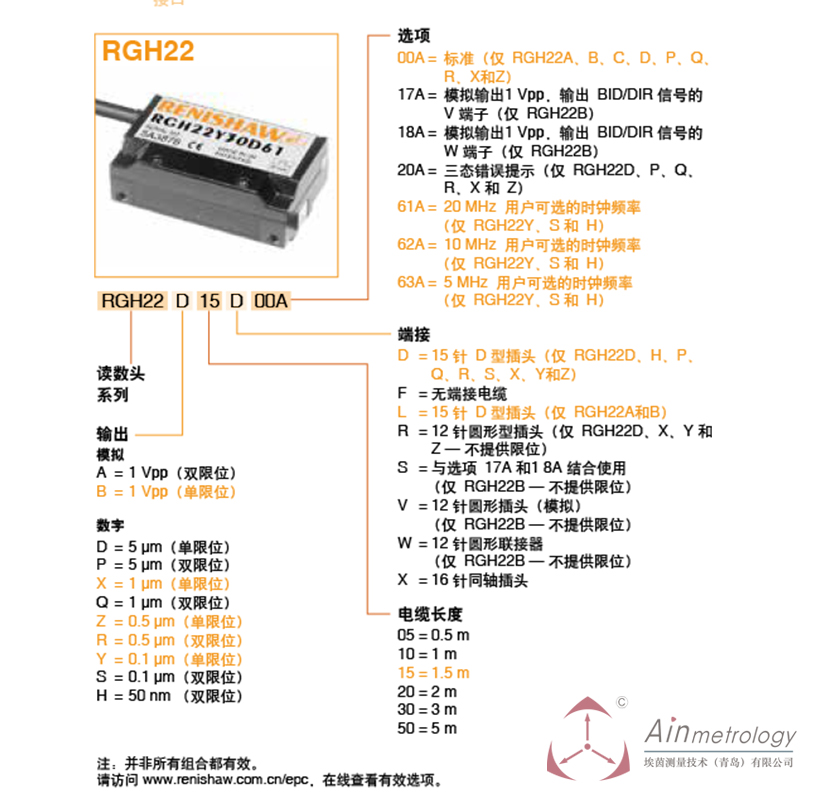•RENISHAW RGH22系列读数头及参数编号

# RENISHAW RGH22系列读数头及参数编号

RGH22R30D00A (0.5μ，双限位)；常规备货，基本全部现货
RGH22S30D61A(0.1μ，双限位)；常规备货，基本全部现货

R位代表的为输出，可选项有

A = 1 Vpp（双限位）
B = 1 Vpp（单限位）

D = 5 μm（单限位）
P = 5 μm（双限位）
X = 1 μm（单限位）
Q = 1 μm（双限位）
Z = 0.5 μm（单限位）
R = 0.5 μm（双限位）
Y = 0.1 μm（单限位）
S = 0.1 μm（双限位）
H = 50 nm （双限位）

30代表电缆长度为3米，3m；可选项有
05 = 0.5 m
10 = 1 m
15 = 1.5 m
20 = 2 m
30 = 3 m
50 = 5 m

D代表端接，可选项有
D = 15 针 D 型插头（仅 RGH22D、H、P、Q、R、S、X、Y和Z）
F = 无端接电缆
L = 15 针 D 型插头（仅 RGH22A和B）
R = 12 针圆形型插头（仅 RGH22D、X、Y 和Z — 不提供限位）
S = 与选项 17A 和1 8A 结合使用（仅 RGH22B — 不提供限位）
V = 12 针圆形插头（模拟），（仅 RGH22B — 不提供限位）
W = 12 针圆形联接器，（仅 RGH22B — 不提供限位）
X = 16 针同轴插头

00A及选项：
00A = 标准（仅 RGH22A、B、C、D、P、Q、R、X和Z）
17A = 模拟输出1 Vpp，输出 BID/DIR 信号的V 端子（仅 RGH22B）
18A = 模拟输出1 Vpp，输出 BID/DIR 信号的W 端子（仅 RGH22B）
20A = 三态错误提示（仅 RGH22D、P、Q、R、X 和 Z）
61A = 20 MHz 用户可选的时钟频率（仅 RGH22Y、S 和 H）
62A = 10 MHz 用户可选的时钟频率（仅 RGH22Y、S 和 H）
63A = 5 MHz 用户可选的时钟频率（仅 RGH22Y、S 和 H）